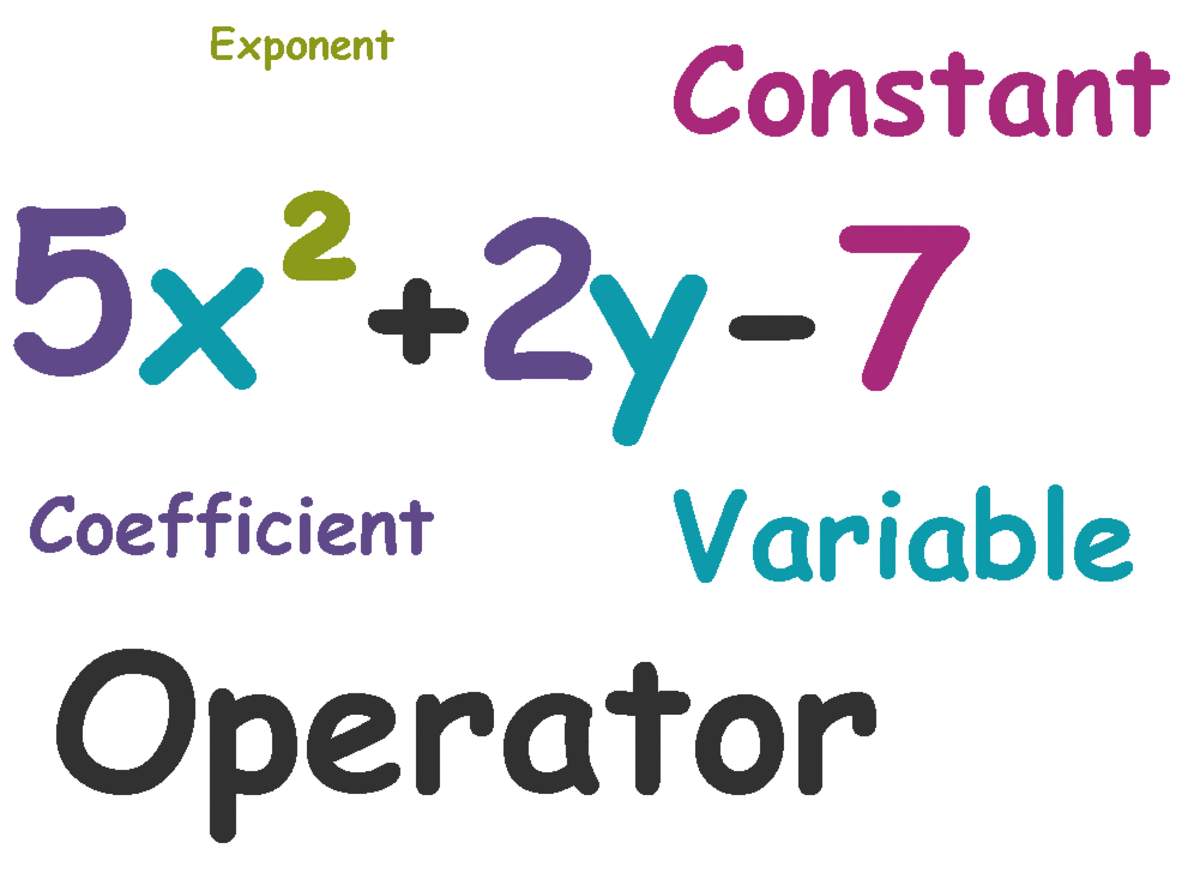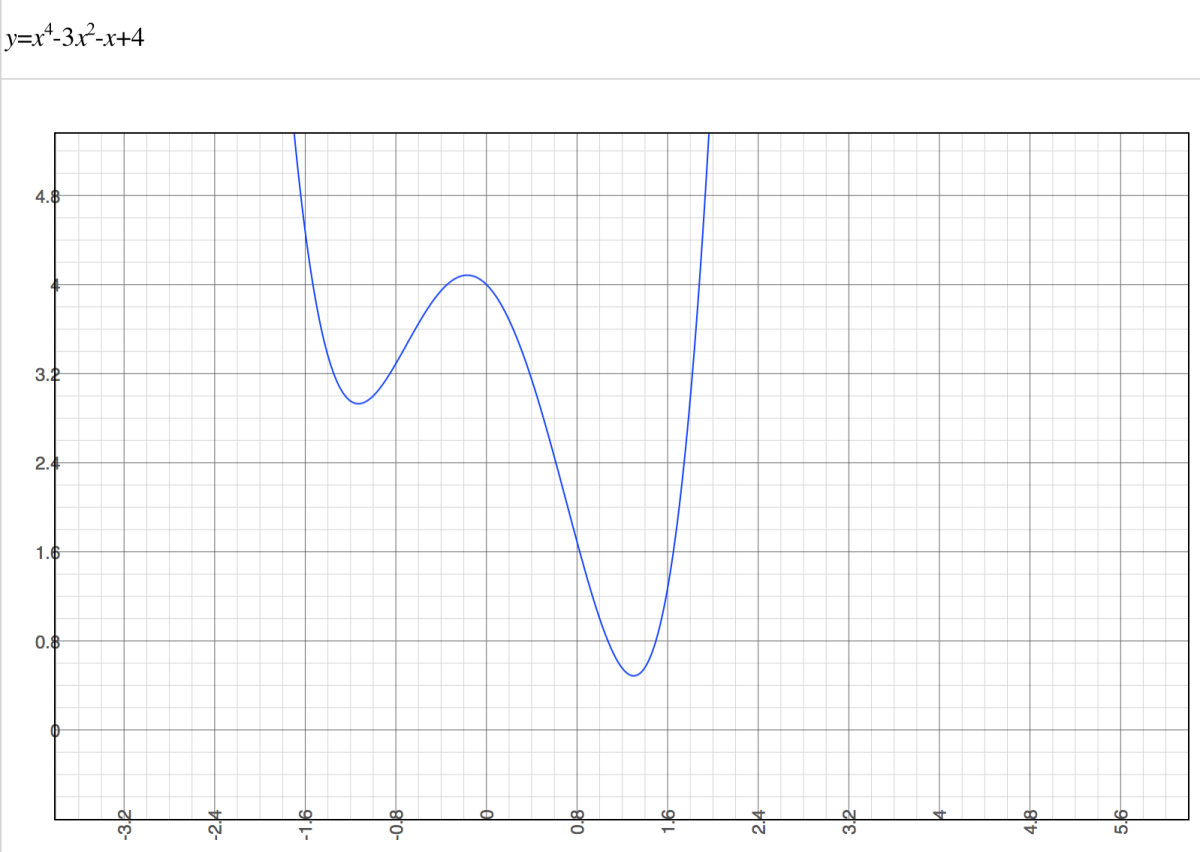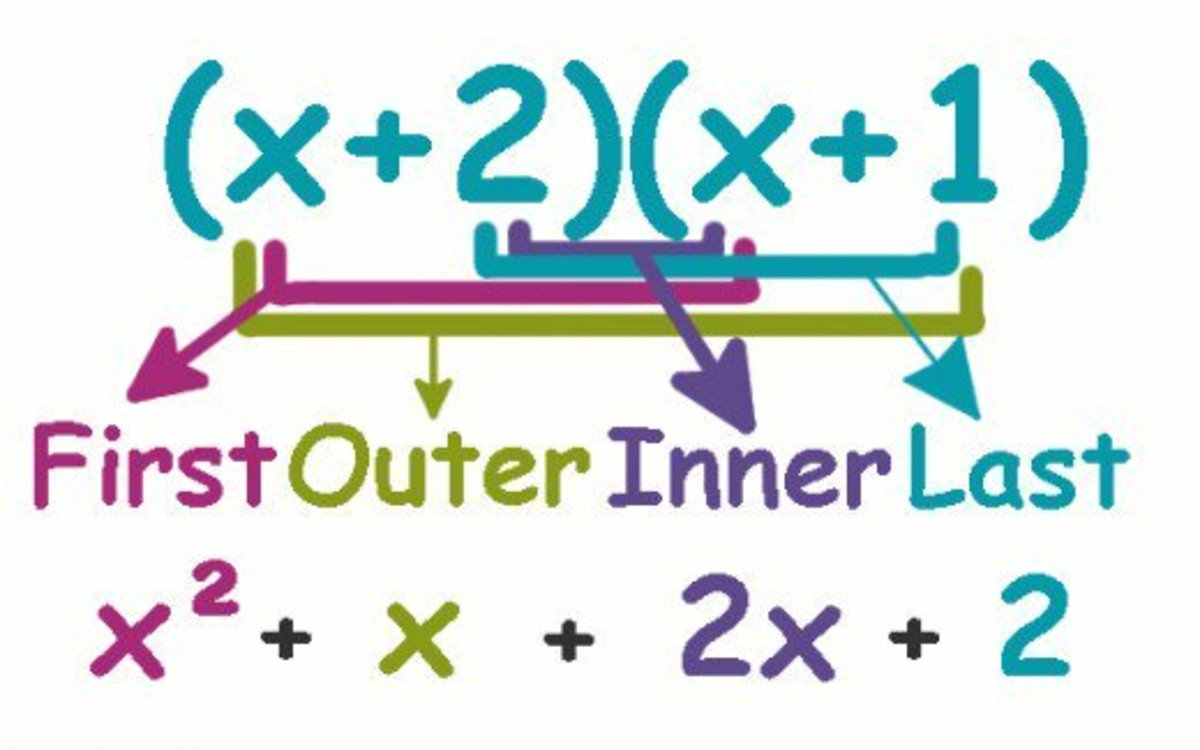# Polynomial Rules: What Defines Polynomials?

Melanie has a BS in physical science and is in grad school for analytics and modeling. She also runs a YouTube channel: The Curious Coder.

## Polynomial Rules

What are the rules for polynomials? The short answer is that polynomials cannot contain the following: division by a variable, negative exponents, fractional exponents, or radicals.

## What is a polynomial?

A polynomial is an expression containing two or more algebraic terms. They are often the sum of several terms having different powers (exponents) of variables.

There are some pretty cool things about polynomials. For example, if you add or subtract polynomials, you get another polynomial. If you multiply them, you get another polynomial.

Polynomials often represent a function. And if you graph a polynomial of a single variable, you'll get a nice, smooth, curvy line with continuity (no holes.)

What does 'polynomial' mean?

The poly in polynomial comes from Greek and means multiple. Nomial, which is also Greek, refers to terms, so polynomial means multiple terms.

## The elements of a polynomialA polynomial can contain variables, constants, coefficients, exponents, and operators.Melanie Shebel

## What Makes Up Polynomials

A polynomial is an algebraic expression made up of two or more terms. Polynomials are composed of some or all of the following:

• Variables - these are letters like x, y, and b
• Constants - these are numbers like 3, 5, 11. They are sometimes attached to variables but are also found on their own.
• Exponents - exponents are usually attached to variables but can also be found with a constant. Examples of exponents include the 2 in 5² or the 3 in x³.
• Addition, subtraction, multiplication, and division - For example, you can have 2x (multiplication), 2x+5 (multiplication and addition), and x-7 (subtraction.)

## Rules: What ISN'T a Polynomial

There are a few rules as to what polynomials cannot contain:

Polynomials cannot contain division by a variable.
For example, 2y2+7x/4 is a polynomial because 4 is not a variable. However, 2y2+7x/(1+x) is not a polynomial as it contains division by a variable.

Polynomials cannot contain negative exponents.
You cannot have 2y-2+7x-4. Negative exponents are a form of division by a variable (to make the negative exponent positive, you have to divide.) For example, x-3 is the same thing as 1/x3.

Polynomials cannot contain fractional exponents.
Terms containing fractional exponents (such as 3x+2y1/2-1) are not considered polynomials.

For example, 2y2 +√3x + 4 is not a polynomial.A graph of a polynomial of a single variable shows nice curvature.Melanie Shebel

## How to find the degree of a polynomial

To find the polynomial degree, write down the terms of the polynomial in descending order by the exponent. The term whose exponents add up to the highest number is the leading term. The sum of the exponents is the degree of the equation.

Example: Figure out the degree of 7x2y2+5y2x+4x2.
Start by adding the exponents in each term.

The exponents in the first term, 7x2y2, are 2 (from 7x2) and 2 (from y2) which add up to four.

The second term (5y2x) has two exponents. They are 2 (from 5y2) and 1 (from x, this is because x is the same as x1.) The exponents in this term add up to three.

The last term (4x2) only has one exponent, 2, so its degree is just two.

Since the first term has the highest degree (the 4th degree), it is the leading term. The degree of this polynomial is four.

For each question, choose the best answer. The answer key is below.

1. What is/are the constant(s) in 3y² + 2x + 5?
• 3
• 2
• 5
• All of the above
2. What is/are the term(s) in 3y² + 2x + 5?
• 3y²
• 2x
• 5
• All of the above
3. What is/are the coefficient(s) in 3y² + 2x + 5?
• 3
• 2
• 5
• Both 3 & 2
4. Which of the following is a variable in 3y² + 2x + 5?
• ²
• x
• 5

1. 5
2. All of the above
3. Both 3 & 2
4. x

## Different types of polynomials

There are different ways polynomials can be categorized. They are often named for the degree of the polynomial and the number of terms it has. Here are some examples:

• Monomials - These are polynomials containing only one term ("mono" means one.) 5x, 4, y, and 5y4 are all examples of monomials.
• Binomials - These are polynomials that contain only two terms ("bi" means two.) 5x+1 and y-7 are examples of binomials.
• Trinomials - These are polynomials that contain three terms ("tri" meaning three.) 2y+5x+1 and y-x+7 are examples of trinomials.
Scroll to Continue

There are quadrinomials (four terms) and so on, but these are usually just called polynomials regardless of the number of terms they contain. Polynomials can have an infinite number of terms, so if you're not sure if it's a trinomial or quadrinomial, you can call it a polynomial.

A polynomial can also be named for its degree. If a polynomial has a degree of two, it is often called a quadratic. If it has a degree of three, it can be called a cubic. Polynomials with degrees higher than three aren't usually named (or the names are seldom used.)

You can do numerous operations on polynomials. Here, the FOIL method for multiplying polynomials is shown.There are a number of operations that can be done on polynomials. Here the FOIL method for multiplying polynomials is shown.Melanie Shebel

## Operations on Polynomials

Now that you understand what makes up a polynomial, it's a good idea to get used to working with them. If you're taking an algebra course, chances are you'll be doing operations on polynomials such as adding them, subtracting them, and even multiplying and dividing polynomials (if you're not already doing so.)

Miltone on May 23, 2020:

Excellent explanation. Thanks.

Naresh on May 12, 2020:

Why polynomials don't have negative exponents? I am not able to find any reason for this.

Ojasva on May 11, 2020:

What is negative exponent or fractional exponent variable called, if not monomial or polynomial

Vin Chauhun from Durban on May 07, 2012:

just looking at those equations caused my brain to breakout into a civil war. :)

Moon Daisy from London on April 18, 2012:

A great hub. I love maths, but I'm a little rusty on the terminology. So thanks!

cardelean from Michigan on April 17, 2012:

Excellent guide. I have a feeling I'll be referring back to it as my kids get a little older! :)

Sondra from Neverland on April 15, 2012:

Melbel I will not take your quiz because I already know I will fail hehe Math never was my thing. Oddly enough my daughter (11) is a math genius and I am going to let her read this tomorrow. She will love it :)

Teresa Coppens from Ontario, Canada on April 15, 2012:

Another great math hub Mel. Very useful for those struggling with these concepts and there are many out there including parents struggling to help their kids in grades 6 to 8 with basic algebra.

Xavier Nathan from Isle of Man on April 15, 2012:

A very nice treatment of this topic and I think you should also create a YouTube channel and make short videos to go with each of your hubs and before long you will have lots of mathematics students following you. Great work.

Jessee R from Gurgaon, India on April 15, 2012:

Nice basic outlay about polynomials... informative

Zulma Burgos-Dudgeon from United Kingdom on April 15, 2012:

I have to confess, I got confused and frustrated after the first paragraph. Math and I don't get on.

But from what I could comprehend this seems to be a good hub and I don't doubt you'll be helping loads of people who maybe didn't understand their instructor's explanation.

Voted up and useful.

Phil Plasma from Montreal, Quebec on April 14, 2012:

Excellent explanation of what a polynomial is.